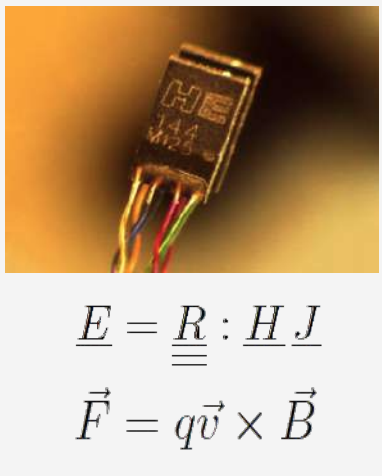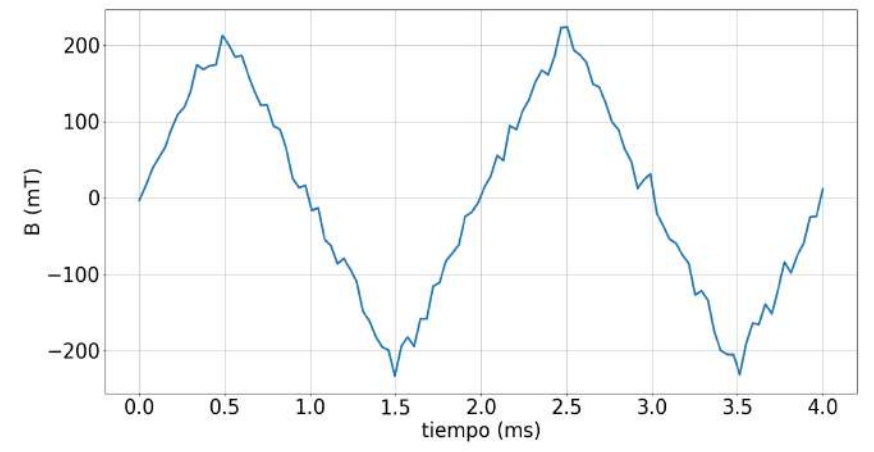# INDUCTOR CHARACTERIZATION THROUGH THE MEASUREMENT OF MAGNETIC FLUX DENSITY

It uses a sensor Technology's HE144 sensor, which has a very linear response to low amplitude fields, as well as very small dimensions. With a thickness of just 0.5 mm, it fits perfectly into the air gap of most inductor designs, therefore being very suitable for these measurements. With this direct measurement, Frenetic's AI can be trained with data that includes more information compared to the measurements of regular electrical parameters.

MEASUREMENT PRINCIPLE

A magnetic field applied to magnetoresistive materials under certain conditions can modify their resistivity. The main physical phenomenon behind this behavior is the Hall effect. If there's a flow of electric current through the material, a Lorentz force caused by the moving electrons within the magnetic field makes the charge carriers accumulate, thus causing a spatial concentration gradient. This provokes the appearance of an electric field and a voltage that can be directly measured. The way the voltage and the magnetic field are related depends on the sensor's material and the angle between the vectors of the acting magnitudes. The analog measured voltage is first amplified and then converted to a digital signal for additional processing, which is then saved to carry the training.Magnetic flux density measurements are useful to check whether the field amplitude predictions are correct. This method can also be applied to study the fringing magnetic flux produced by the air gap reluctance. Under certain assumptions, a reduction in its density with respect to the one calculated for the core can be an indication of the existence of this effect.CONCLUSIONS

The characterization of inductors by measuring the magnetic flux density is useful to correlate other measured parameters. Also, the Fringing flux in the air gap can be determined, thus obtaining data to ameliorate the model that describes the inductor behavior. The data acquired through this process is used to train machine learning models and Frenetic's AI to predict phenomena that analytical models cannot.